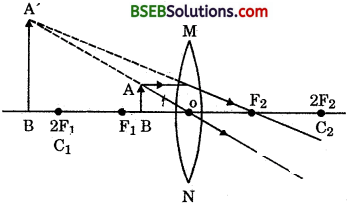# Bihar Board Class 10 Science Solutions Chapter 10 Light Reflection and Refraction

## BSEB Bihar Board Class 10 Science Solutions Chapter 10 Light Reflection and Refraction

Bihar Board Class 10 Science Solutions Chapter 10 Light Reflection and Refraction Textbook Questions and Answers, Additional Important Questions, Notes.

### Bihar Board Class 10 Science Chapter 10 Light Reflection and Refraction InText Questions and Answers

Intext Questions (Page 168)

Question 1.
Define the principal focus of a concave mirror.
The point on principal axis where beam of rays parallel to principal axis meet after reflection is called principal focus.

Question 2.
The radius of curvature of a spherical mirror is 20 cm. What is its focal length ?
We know that
R = 2f.
Here, R = 20 cm.
∴ f = $$\frac {20}{2}$$ = 10 cm
Focal length of concave mirror = 10 cm k

Question 3.
Name a mirror that gives an erect and enlarged image of an object.
The name of mirror is concave mirror.

Question 4.
Why do we prefer a concave mirror as a rear view mirror in vehicles ? .
Convex mirror is used as rear view mirror in vehicle because its aperture is large and it always forms virtual, erect and full size diminished image of even far objects.Intext Questions (Page 171)

Question 1.
Find the focal length of a convex mirror whose radius of curvature is 32 cm.
Given R = 32 cm
R = 2f
f = 16 cm

Question 2.
A concave mirror produces three times magnified (enlarged) real image of an object placed at 10 cm in front of it. Where is the image located ?
m = – $$\frac {υ}{u}$$ (real)
or – 3 = – $$\frac {υ}{u}$$
or 3u = υ
u = – 10 cm (given)
υ = – 30 cm (as υ = 3u)
∴ Image is formed at a distance of 30 cm from pole in front of convex mirror.

Intext Questions (Page 176)

Question 1.
A ray of light travelling in air enters obliquely into water. Does the light ray bend towards the normal or away from the normal ? Why ?
It will bend towards the normal because its velocity will decrease as it will enter in water. .

Question 2.
Light enters from air to glass having refractive index 1.50. What is the speed of light in the glass ? The speed of light in vacuum is 3 x 108 ms-1.C = velocity of light in air,
υg = velocity of light in glass.

Question 3.
Find out from Table 10.3, the medium having highest optical density. Also find the medium with lowest optical density. –
Medium having highest optical density = Diamond. Medium having largest optical density = air = 1.003.

Question 4.
You are given kerosene, turpentine and water. In which of these does the light travel fastest ? Use the information given in Table 10.3.
Light travels faster in water as its refractive index is higher than kerosene oil and turpentine oil.

Question 5.
The refractive index of diamond is 2.42. What is the meaning of this statement ?
Diamond is the optically most dense medium and the value of speed of light in diamond is very less.Intext Questions (Page 184)

Question 1.
Define 1 dioptre of power of a lens.
When the focal length of a lens is one metre, then the power of the lens is called 1dioptre.

Question 2.
A convex lens forms a real and inverted image of a needle at a distance of 50 cm from it. Where is the needle placed in front of the convex lens if the image is equal to the size of the object ? Also, find the power of the lens.
Here image distance = + 50 cm
υ = 50 cm.
m = 1
p = $$\frac {1}{f}$$ = ?
m = $$\frac {υ}{u}$$
1 = $$\frac {υ}{u}$$
υ = u
∴ υ = – 50 cm
∴ Object distance = 50 cm
Now, $$\frac {1}{υ}$$ – $$\frac {1}{u}$$ = $$\frac {1}{f}$$
⇒ $$\frac {1}{50}$$ – $$\frac {1}{-50}$$ = $$\frac {1}{f}$$
⇒ $$\frac {1 + 1}{50}$$ = $$\frac {1}{f}$$
∴ f = 25 cm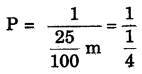p = 4 dioptre

### Bihar Board Class 10 Science Chapter 10 Light Reflection and Refraction Textbook Questions and Answers

Question 1.
Which one of the following materials cannot be used to make a lens ?
(a) Water
(b) Glass
(c) Plastic
(d) Clay.
(d) Clay.

Question 2.
Hie image formed by a concave mirror is observed ; to be virtual, erect and larger than the object. Where should be the position of the object ?
(а) between the principal focus and the centre of curvature
(b) at the centre of curvature
(c) beyond the centre of curvature .
(d) between the pole of mirror and its principal focus.
(d) between the pole of the mirror and its principal focus.

Question 3.
Where should an object be placed in front of a convex lens to get a real image of the size of the object ?
(a) At the principal focus of the lens
(b) At twice the focal length
(c) At infinity
(d) Between the optical centre of the lens and its; principal focus.
(c) At infinity.Question 4.
A spherical mirror and a thin spherical lens have ” each a focal length of – 15 cm. The mirror and the lens are likely to be
(а) Both are concave
(b) Both are convex
(c) The mirror is concave and the lens is convex:
(d) The mirror is convex, but the lens is concave.
(a) Both are concave.

Question 5.
No matter how far you stand from a mirror, your image appears erect. The mirror is likely to be
(а) plane only
(b) concave only
(c) convex only
(d) either plane or convex.
(d) Either plane or convex.

Question 6.
Which of the following lenses would you prefer to use while reading small letters found in a dictionary ?
(а) A convex lens of focal length 50 cm
(b) A concave lens of focal length 50 cm
(c) A convex lens of focal length 5 cm
(d) A concave lens of focal length 5 cm.
(c) A convex lens of focal length 5 cm.

Question 7.
We wish to obtain an erect image of an object, using a concave mirror of focal length 15 cm. What should be the range of distance of the object from the mirror? What is the nature of the image ? Is the image larger or smaller than the object ? Draw a ray diagram to show the image formation in this case.
Range of distance of the object is 0 to 15 cm (i.e., less than 15 cm) in front of mirror from pole of mirror.
(i) Nature of image : Virtual and erect.
(ii) Image is larger than object.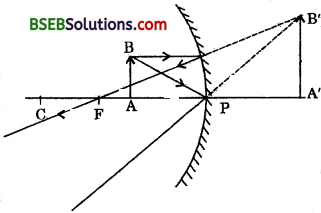Question 8.
Name the type of mirror used in the following situations:
(а) Head lights of a car
(b) Side /rear-view mirror of vehicle
(c) Solar furnace.
(a) Concave mirror.
(c) Concave mirror.
(b) Convex mirror.

Question 9.
One half of a convex lens is covered with a black paper. Will this lens produce a complete image of the object ? Verify your.answer experimentally. Explain your observations.
Yes, the lens will produce the full size image of the object.
Verification : Taken a convex lens one half covered with a black paper. Put an object before it and obtained a image on the screen by adjustment. Observed that the lens produces complete of the object.

Question 10.
An object 5 cm in length is held 25 cm away from a converging lens of focal length 10 cm. Draw the ray diagram and find the position, size and the nature of the imaged formed.
h1 = 5 cm, u = -25 cm, f = + 10 cm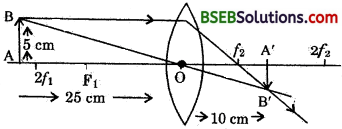$$\frac {1}{υ}$$ – $$\frac {1}{u}$$ = $$\frac {1}{f}$$
$$\frac {1}{υ}$$ – $$\frac {1}{-25}$$ = $$\frac {1}{10}$$
$$\frac {1}{υ}$$ = $$\frac {1}{10}$$ – $$\frac {1}{25}$$
= $$\frac {5 – 2}{50}$$ = $$\frac {3}{50}$$
υ = $$\frac {50}{3}$$
υ = 16.66 cm
Image is for 16.66 cm (ray 16.7 cm) from the lens on other side, i.e., behind the lens. Image is real and inverted.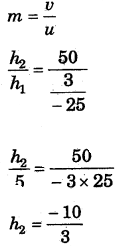h2 = – 3.33 cm
Image is inverted and a height of 3.33 cm.Question 11.
A concave lens of focal length 15 cm forms an image 10 cm from the lens. How far is the object placed from the lens ? Draw the ray diagram.
f = – 15 cm, υ = – 10 cm, u = ?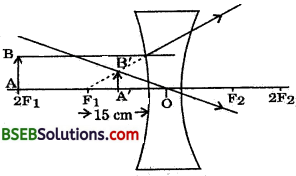$$\frac {1}{υ}$$ – $$\frac {1}{u}$$ = $$\frac {1}{f}$$
⇒ $$\frac {1}{-10}$$ – $$\frac {1}{u}$$ = $$\frac {1}{-15}$$
– $$\frac {1}{10}$$ + $$\frac {1}{15}$$ = $$\frac {1}{u}$$
$$\frac {1}{u}$$ = $$\frac {-3 + 2}{30}$$
u = – 30 cm
Object is placed at a distance of 30 cm from concave lens.

Question 12.
An object is placed at a distance of 10 cm from a convex mirror of focal length 15 cm. Find the position and nature of the image.
f = 15 cm, u = – 10 cm, υ = ?
We know for spherical mirror
$$\frac {1}{u}$$ + $$\frac {1}{υ}$$ = $$\frac {1}{f}$$
– $$\frac {1}{-10}$$ + $$\frac {1}{υ}$$ = $$\frac {1}{15}$$
$$\frac {1}{υ}$$ = $$\frac {1}{15}$$ + $$\frac {1}{10}$$
$$\frac {1}{υ}$$ = $$\frac {2 + 3}{30}$$
$$\frac {1}{υ}$$ = $$\frac {5}{30}$$
υ = 6 cm
Image is formed 6 cm behind the mirror. Image is virtual and erect.

Question 13.
The magnification produced by a plane mirror is + 1. What does this mean ?
The, positive sign means image formed a plane mirror is virtual and erect the size of image is equal to the size of object.

Question 14.
An object 5.0 cm in length is placed at a distance of 20 cm in front of a convex mirror of radius of curvature 30 cm. Find the position of the image, its nature and size.
h1 = 5 cm,
$$\frac {R}{2}$$ = f = $$\frac {30}{2}$$ = + 15 cm, u = -20cm υ = ? h2 = ?
$$\frac {1}{υ}$$ + $$\frac {1}{u}$$ = $$\frac {1}{f}$$
⇒ $$\frac {1}{υ}$$ + $$\frac {1}{-20}$$ = $$\frac {1}{+15}$$
$$\frac {1}{υ}$$ = $$\frac {1}{15}$$ + $$\frac {1}{20}$$ = $$\frac {4 + 3}{60}$$
υ = $$\frac {60}{7}$$ cm = 8.57 cm
The screen should be kept at a distance of 54 cm in front of mirror.
m = $$\frac{h_{2}}{h_{1}}=\frac{-v}{u}$$
$$\frac{h_{2}}{5 \mathrm{~cm}}=\frac{8.57}{20}$$
or h2 = $$\frac{3.57 \times 5}{20}$$
Size of image = 2.175 (say 2.2 cm)
Image is virtual, erect and of height 2.2 cm reduced.Question 15.
An object of size 7.0 cm is placed at 27 em in front of a concave mirror of focal length 18 cm. At What distance from the mirror should a screen be placed, so that a sharp focused image can be obtained? Find the size and the nature of the images
h1 = 5.0 cm, u = -27cm, f = -l8 cm υ = ? h1 = ?
We know –
$$\frac {1}{u}$$ + $$\frac {1}{υ}$$ = $$\frac {1}{f}$$
⇒ $$\frac {1}{-27}$$ + $$\frac {1}{υ}$$ = $$\frac {1}{-18}$$
$$\frac {1}{υ}$$ = $$\frac {- 1}{18}$$ + $$\frac {1}{27}$$
= $$\frac {- 3 + 2}{54}$$
$$\frac {1}{υ}$$ = –$$\frac {1}{54}$$
υ = – 54 cm
The screen should be kept at a distance of 54 cm in front of mirror.
m = $$\frac{h_{2}}{h_{1}}=\frac{-v}{u}$$
$$\frac{h_{2}}{7 \mathrm{~cm}}=-\frac{(-54)}{(-27)}$$
$$\frac{h_{2}}{7 \mathrm{~cm}}$$ = 2
h2 = 2 x 7
h2 = 14 cm
Height of image is 14 cm, the image is real and inverted.

Question 16.
Find the’focal length of a lens of power – 2.0 D. What type of lens is this ?
p = $$\frac {1}{f}$$
– 20 = $$\frac {1}{f}$$
f = $$\frac {-1}{2}$$ m
f = $$\frac {-1}{2}$$ x 100 cm
f = – 50 = – 0.50 m
The lens is concave lens.

Question 17.
A doctor has prescribed a corrective lens of power + 1.5 D. Find the focal length of the lens. Is the prescribed lens diverging or converging ?
p = $$\frac {1}{f}$$
1.5 = $$\frac {1}{f}$$
f = $$\frac {1}{1.5}$$ m = $$\frac {10}{15}$$ m
= $$\frac {2}{3}$$ m = + 0.67 m
Focal length of the lens is + 0.67 m. The prescribed lens is converging in nature.### Bihar Board Class 10 Science Chapter 10 Light Reflection and Refraction Textbook Activities

Activity 10.1 (Page 161)

Take a large shining spoon. Try to view your face in its curved surface.

Question 1.
Do you get the image ? Is it smaller or larger ?
Yes. The image is smaller.

Question 2.
Move the spoon slowly away from your face. Observe the image. How does it change ?
Its size becomes smaller.

Question 3.
Reverse the spoon and repeat the activity. How does the image look like now ?
Earlier, image was inverted. Now the image is erect.

Question 4.
Compare the characteristics of the image two surfaces.
Inner surface
(i) The image is sometimes inverted, some times erect.
(ii) Image size is some times smaller and sometimes larger.

Outer surface :
Image is always erect.
Image is always smaller.Question 5.
Why do we see our image in shining spoon ?
From the surface of shining spoon reflection takes place and hence it acts as a mirror. So we see our image in it.

Question 6.
What type of mirror would be the inward and outward shining surface of spoon ?
Outward curved surface acts as convex mirror and inward curved surface acts as concave mirror of shining spoon.

Question 7.
How does image change when the face is slowly moved away from shining spoon ?
In case of outward shining surface of spoon : “As face is moved away the size of image decreases but image remain erect.” In case of inward shining surface of spoon : “As face is moved away the size of image decreases but image is inverted.”

Activity 10.2 (Page 162)

CAUTION : Do not look at the Sun directly or even into a mirror reflecting sunlight. It may damage your eyes.

• Hold a concave mirror in your hand and direct its reflecting surface towards the Sun.
• Direct the light reflected by the mirror on to a sheet of paper held close to the mirror.
• Move the sheet of paper back and forth gradually until you find on the paper sheet a bright, sharp spot of light.
• Hold the mirror and the paper in the same position for a few minutes.

Question 1.
What do you observe ?
The paper starts burning. All the heat rays from sun are concentrated at that point.

Question 2.
Why should not we see the sun directly or even into a mirror reflecting sunlight ?
Because it may damage the eye.

Question 3.
What happens when the rays of sun are focused at a point by using concave mirror?
The paper starts burning.

Question 4.
Name the point on paper where sharp image of sun is formed by focussing the reflected sun ray with the help of concave mirror.
Focus of concave mirror.

Question 5.
What is called the distance between focus and the mirror ?
It is called focal length.Activity 10.3 (Page 163)

You have already learnt a way of determining the focal length of a concave mirror. In activity 10.2, you have seen that the sharp bright spot of light you got on the paper is, in fact, the image of the Sun. It was a tiny, real, inverted image. You got the approximate focal length of the concave mirror by measuring the distance of the image from the mirror.

• Take a concave ipirror. Find out its approximate focal length in the way described above. Note down the value of focal length. (You can also find it out by obtaining image of a distant object on a sheet of paper).
• Mark a line on a table with a chalk. Place the concave mirror on a stand. Place the stand over the line such that its pole lies over the line.
• Draw with a chalk two more lines parallel to the previous line such that the distance between any two successive lines is equal to the focal length of the mirror. These lines will now correspond to the positions of the points P, F and C, respectively. Remember: For a spherical mirror of small aperture, the principal focus F lies mid-way between the pole P and the centre of curvature C.
• Keep a bright object, say a burning candle, at a position far
beyond C. Place a paper screen and move it in front of the mirror till you obtain a sharp bright image of the candle flame on it.
• Observe the image carefully. Note down its nature, position
and relative size with respect to the object size. ,
• Repeat the experiment by placing the candle (a) just beyond C, (b) at C, (c) between F and C, (d) at F, and (e) between P and F.
• In one of the cases, you may not get the image on the screen. Identify the position of the object in such a case.
• Then, look for its virtual image in the mirror itself.

Question 1.
Note down and tabulate your observations.
Image formation by a concave mirror for different positions of the object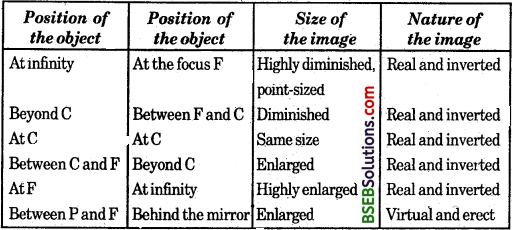Questions Based on the Activity

Question 2.
What is relation between focal length and radius of curvature ?
Radius of curvature is twice the focal length.

Question 3.
What is the nature of image when object is placed v for beyond C?
The image of object is formed at focus and it is real, inverted and highly diminished.

Question 4.
What is the nature and position of image if the object is kept just behind the centre of curvature ?
The image is formed in between focus and centre of curvature. It is real, inverted and smaller than object.

Question 5.
Draw the ray diagram of formation of image when object is kept at centre of curvature in front of a concave mirror.Question 6.
In which case the image formed by concave mirror is not obtained on the screen ?
The imagery concave mirror is not obtained on screen when the object is kept between pole and focus of concave mirror.

Question 7.
In which case the image of object by concave mirror can be obtained on the screen ? . ,
The image of object is obtained by concave mirror when the object is kept beyond focal length of mirror.Activity 10.4 (Page 166)

Question 1.
Draw neat ray diagrams for each position of the object shown in Table 10.1 of your book. You may take any two of the rays mentioned in the previous section for locating the image.Question 2.
Compare your diagram with those given in Fig. 10.7 of your book.
They are exactly alike.

Question 3.
Describe the nature, position and relative size of the image formed in each case. Tabulate the results in a convenient format.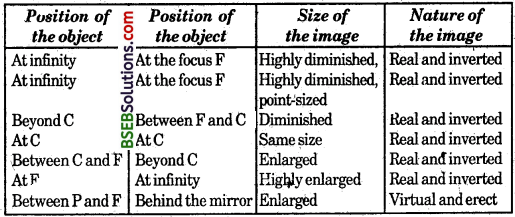Activity 10.6 (Page 167)

• Observe the image of a distant object, say a distant tree, in a plane mirror.

Question 1.
Could you see a full-length image ?
No.

Question 2.
Try with plane mirrors of different size ? Did you see the entire object in the image ?
No, I didn’t.

Question 3.
Repeat this activity with a concave mirror. Did the mirror show full length image of the object ?
No.

Question 4.
Now try using a convex mirror. Did you succeed? Explain your observations with reason. ,
Yes, now I succeeded to see the full-length image of that distant object. Convex mirror always form diminished, erect’ and virtual image of any object. That is why, a distant object can be seen clearly in a convex mirror.Activity 10.7 (Page 172)

• Place a coin at the bottom of a bucket filled with water.

Question 1.
With your eye to a side above water, try to pick up the coin in one go. Did you succeed in picking up the coin ?
No.

Question 2.
Repeat the activity. Why did you not succeed in doing it in one go ?
Because, the coin appeared raised-

Question 3.
Do it yourself.

Questions Based on the Activity

Question 4.
The image of coin in a water containing bucket appears raised above from its original position. Why?
As ray coming from coin travels from water to air it undergoes refraction arid its apparent image is raised up from its real position. So its original position appears to he raised above.

Question 5.
Can you pick up a coin in a water containing bucket in one go? Why?
No. Because we see the apparent position of coin due to refraction when we see it to a side above water. The coin is not actual at the apparent ppsition.

Activity 10.8 (Page 172)

Question 1.
Does the coin becomes visible again from your position ? How could this happen ?
Yes. The coiri becomes visible again on passing water into bowlybecause due to refraction Of light the position of coin is raised above than its actual position and hence coin appears.

Activity 10.9 (Page 172)

• Draw a thick straight line in ink, over a sheet of white paper placed on a table.
• Place a glass slab over the line in such a way that one of its edges makes an angle with the line.

Question 1.
Look at the portion of the line under the slab from the sides. What do you observe ? Does the line under “the glass slab appear to be bent at the edges ?
Yes. It is due to the refraction of light.

Question 2.
Next, place the glass slab such that it is normal to the line. What do you observe now ? Does the part of the line under the glass slab appear bent ?
Now, the line is straight and there is no bending because for normal ray of light, there is no refraction.

Question 3.
Look at the line from the top of the glass slab. Does the part of the line, beneath the slab, appear to be raised ? Why does this happen ?
Yes, it is also because of the refraction. Due to this, the apparent image of object is raised above from its actual point.Activity 10.10 (Page 173)

Questions Based on the Activity

Question 1.
What happens when light ray enters to glass-slab?
The light ray undergoes refraction and due to decrease in its veìocity it bends towards normal.

Question 2.
What happens when light ray emerges again in air from glass ?
As ray of light emerges in air due to increase in velocity the light ray bends away from normal.

Question 3.
What is the perpendicular distance between direction of emergent ray and direction of incidence ray called ?
Lateral displacement.

Question 4.
What is relation between angle of incidence and angle of emergence if the medium of incident ray and emergent ray is same ?
Angle of incidence = Angle of emergence.

Activity 10.11 (Page 177)

Question 1.
What happened to the paper ? Why ?
The paper was burnt. The lens focussed the sunrays at a point. Sunrays have energy.

Activity 10.12 (Page 178)

• Take a convex lens. Find its approximate focal length in a \yay described in Activity 10.11.
• Draw five parallel straight lines, using chalk, on a long table such that the distance between the successive lines is equal to the focal length of the lens.
• Place the lens on a lens stand. Place it on the central line such that the optical centre of the lens lies just over the line.
• The two lines on either side of the lens correspond to F and 2F of the lens respectively. Mark them with appropriate letters such as 2F1; F1; F2 and 2F2, respectively.
• Place a burning caridie, far beyond 2F1 to the left. Obtain a clear sharp image on a screen on the opposite side of the lens.
• Note down the nature, position and relative size of the image.
• Repeat this activity by placing object just behind  2F1 between F1 and 2F1
• at F1; between F1 and 0. Note down and tabulate your observations.Activity 10.13 (Page 179)

• Take a concave lens. Place it on a lens stand.
• Place a burning candle on one side of the lens.
• Look- through the lens from the other side and observe the image. Try to get the image on a screen, if possible. If not,’ observe the image directly through the lens.

Question 1.
Note down the nature, relative size and approximate position of the image.
The images is formed between focus Fx and optical centre O. It is diminished, virtual and erect.

Question 2.
Move the candle away from the lens. Note the change in the size of the image.
It is diminished.### Bihar Board Class 10 Science Chapter 10 Light Reflection and Refraction NCERT Exemplar Problems

Question 1.
Identify the device used as a spherical mirror or lens in the following cases, when the image formed is virtual and erect in each case.
(а) Object is placed between device and its focus, image formed is enlarged and behind it.
(b) Object is placed between the focus and device, image formed is enlarged and on the same side as that of the object.
(c) Objeet is placed between infinity and device, image formed is diminished and between focus and optical I centre on the same side as that of the object.
(d) Object is placed between infinity and device, image formed is diminished and between pole and focus, behind it.
(a) concave mirror
(b) convex lens
(c) concave lens
(d) convex mirror

Question 2.
Why does a light ray incident on a rectangular glass slab immersed lit any medium emerges parallel to itself? Explain using a diagram.
Let ABCD is a glass slab when light ray EF strikes the face AB of the glass, it passes from air (rarer) to glass (denser)
due to refraction and moves towards the normal, when this light ray strikes the face CD of the slab, it now passes from glass (denser) to air (rarer) due to refraction and moves away from the normal. When angles and i1 and r2 are measured and EF is extended and compared with the emergent ray, it is found that the incident ray and emergent ray are parallel to each other.Question 3.
A pencil when dipped in water in a glass tumbler appears to be bent at the interface of air and water. Will the pencil appear to be bent to the same extent, if instead of water we use liquids like, kerosene or turpentine. Support your answer with reason.
No. Bending will be different in different liquids since velocity of light at the interface separating two media depends on the relative refractive index of the medium.Question 4.
How is the refractive index of a medium related to the speed of light? Obtain an expression for refractive index of a medium with respect to another in terms of speed of light in these two media?
If c is the speed of light in air and v is the speed of light in the medium, then, the refractive index of the medium n. is given byObtaining an expression for refractive index of a medium with respect to another in terms of speed of light in these two media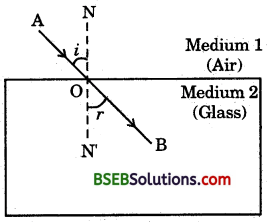Consider a ray of light travelling from medium 1 into medium 2, as shown in the figure. Let v1be the speed of light in medium 1 and v2 be the speed of light in medium 2. The refractive index of medium 2 with respect to medium 1 is given by the ratio of the speed of light in medium 1 and the speed of light in medium 2. This is usually represented by the symbol n21 This can be expressed in an equation form asQuestion 5.
Refractive index of diamond with respect to glass is 1.6 and absolute refractive index of glass is 1.5. Find’ out the absolute refractive index of diamond.
Let –
υg represents the velocity of light in glass
υd represents the velocity of light in diamond
ng represents the absolute refractive index of glass
ndrepresents the absolute refractive index of diamond
c represents velocity of light in air
ndgrepresents refractive index of diamond with respect to glass
Now, according to given information: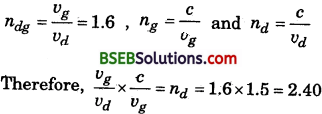Question 6.
A convex lens of focal length 20 cm can produce a magnified virtual as well as real image. Is this a correct statement? If yes, where shall the object be placed in each case for obtaining these images?
We know that a convex lens produces a magnified, virtual and erect image when the object is placed between the focus F1 and optical centre O and it produces a magnified, real and inverted image when the object is placed between F. and 2Fr ‘
Thus, the given statement is correct if the object is placed within 20 cm from the lens in the first case and between 20 cm and 40 cm in the second case.

Question 7.
Sudha finds out that the sharp image of the window pane of her science laboratory is formed at a distance of 15 cm from the lens. She now tries to focus the building visible to her Outside the window instead of the window, pane without disturbing the lens. In which direction will she move the screen to obtain a sharp image of the building? What is the approximate focal length of this lens?
Sudha should move the screen towards the lens so as to obtain a clear image of the building. The approximate focal length of this lens will be 15 cm.Question 8.
How are power and focal length of a lens related? You are provided with two lenses of focal length 20 cm and 40 cm respectively. Which lens will you use to obtain more convergent light?
Let P represents power and/’represents the focal length of a lens then relation between P and f‘of a lens is mathematically
represented as : p = $$\frac {1}{f}$$, p ∝ $$\frac {1}{f}$$. Power of a lens is inversely
proportional to its focal length therefore, lens having focal length of 20 cm will provide more convergence.

Question 9.
Under what condition in an arrangement of two * plane mirrors, incident ray and reflected ray will always be parallel to each other, whatever may be angle of incidence. Show the same with the help of diagram.
When two plane mirrors are placed at right angle to each other then the incident and reflected rays will always be parallel to each other.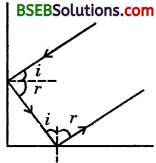Question 10.
Draw a ray diagram showing the path of rays of light when it enters with oblique incidence (i) from air into water; (£/) from water into air.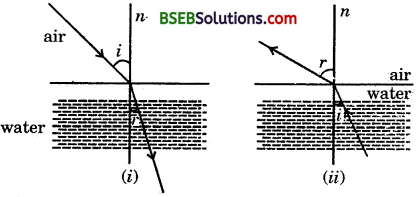Question 11.
Draw ray diagrams showing the image formation by a concave mirror when an object is placed
(a) between pole and focus of the mirror
(b) between focus and centre of curvature of the mirror
(c) at centre of curvature of the mirror
(d) a little beyond centre of curvature of the mirror
(e) at infinity
Ray diagrams showing the image formation by a concave mirror.
(a) When an object is placed between pole and focus of the mirror(b) When an object is placed between focus and centre of curvature of the mirror.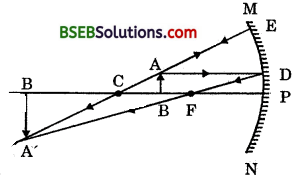(c) When an object is placed at centre of curvature of the mirror.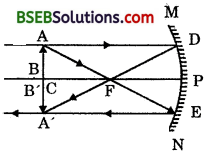(d) When an object is placed a little beyond the centre of curvature of the mirror.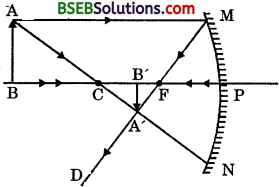(e) When an object is placed at infinity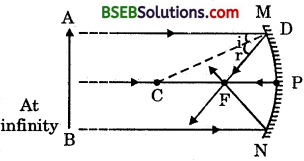The table given below describes the nature, position and relative size of the image formed in each case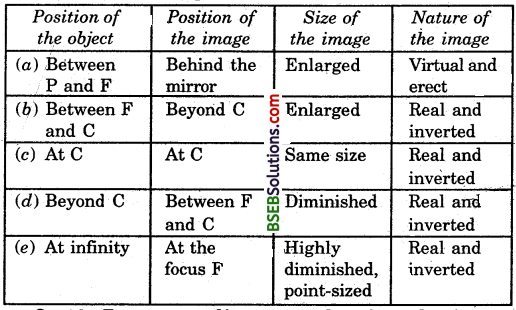Question 12.
Draw ray diagrams showing the image formation by a convex lens when an object is placed
(а) between optical centre and focus of the lens
(b) between focus and twice the focal length of the lens
(c) at twice the focal length of the lens
(d) at infinity
(e) at the focus of the lens
Ray diagrams showing the image formation by a convex lens :
(a) When an object is placed between optical centre and focus of the lens.(b) When an object is placed betweeñ focus and twice the focal length of the lens.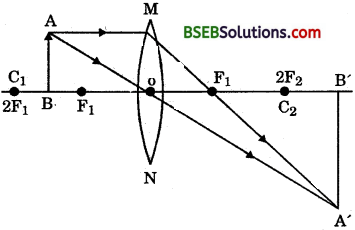(c) When an object is placed at twice the focal length of the lens.(d) When an object is placed at infinity(e) When an object is at the focus of the lens.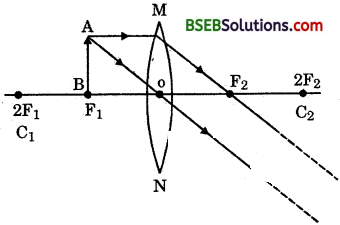The table given below describes the nature, position and relative size nf the image formed in each case.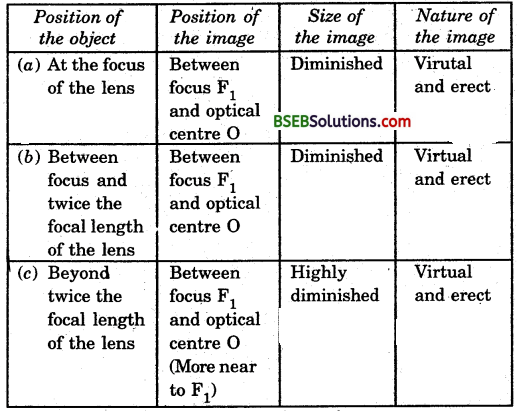Question 13.
Write laws of refraction. Explain the same with the help of ray diagram, when a ray of light passes through a rectangular glass slab.
Laws of refraction are :

1. The incident ray, the refracted ray and the normal to the interface of two transparent media at the point of incidence, all lie in the same plane.
2. The ratio of sine of angle of incidence to the sine of angle of refraction is a constant, for the light of a given colour and for the given pair of media.
3. This law is also known as Snell’s law of refraction.
4. If f is the angle of incidence and r is the angle of refraction, then,This constant value is called the refractive index of the second medium with respect to the first.In the ray diagram shown here, EO, the incident ray is changing its direction at O as it has entered from a rarer medium to a denser medium, i.e., from air to glass. Here, the light ray bends towards the normal. Thus, OCT is the refracted ray. At O’, the light ray enters from glass to air, i.e., from a denser medium to a rarer medium. The light here bends away from the normal.

Thus the ray 00′, changes its direction at O’, OH is the emergent ray which is parallfeTto the direction of the incident ray. It is clear from the ray diagram that incident ray EO’, the refracted ray 00′, and the normal NN’, to the interface of two transparent media at the point of incidence, all lie in the same plane. It is the first law of refraction. Now, if we compare the angle of incidence with the angle of refraction at both refracting surfaces AB and CD and take ratio of the sine of these angles, we find that –which is the second law of refraction.Question 14.
Draw ray diagrams showing the image formation by a concave lens when an object is placed
(a) at the focus of the lens
(b) between focus and twice the focal length of the lens
(c) beyond twice the focal length of the lens
Ray diagrams showing the image formation by a concave

(a) When an object is placed at the focus of the lens.(b) When the object is placed between focus and twice the focal length of the lens.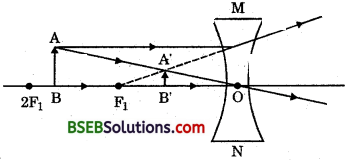(c) When the object is placed beyond twice the focal length of the lens.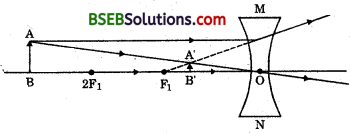(d) The table given below describes the nature, position and relative size of the image formed in each case.Question 15.
Draw ray diagrams showing the image formation by a convex mirror when an object is placed
(а) at infinity
(b) at finite distance from the mirror
Ray diagrams showing the image formation by a convex mirror :
(a) When an object is placed at infinity.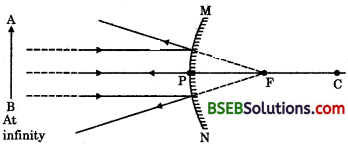(b) When an object is at finite distance from the mirror.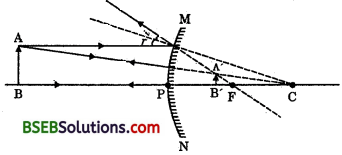(c) The table given below shows the nature, position and relative size of the image formed by a convex mirror.The table given below shows the nature, position and relative size of the image formed by a convex mirror.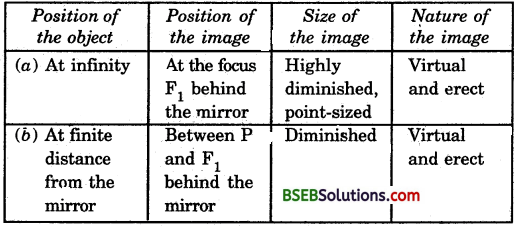Question 16.
The image of a candle flame formed by a lens is obtained on a screen placed on the other side of the lens. If the image is three times the size of the flame and the distance between lens and image is 80 cm, at what distance should the candle be placed from the lens? What is the nature of the image at a distance of 80 cm and the lens ?
It is given that the image of a candle flame is obtained on a screen. Thus, the image formed is real and for a real image the magnificatioh is taken as negative.
∴ M = – 3 [∴ Image formed is three times the size of the flame]
Now, M = $$\frac {υ}{u}$$
⇒ -3 = $$\frac {υ}{u}$$
The image is formed at a distance of 80 cm from the lens on the other side of the lens.
∴ υ = 80 cm
From the above equation
– 3 = $$\frac {80}{u}$$
⇒ u = $$\frac {80}{-3}$$ = 26.66 cm
∴ The candle should be placed at a distance of approx. 27 cm from the lens.
As the image formed is enlarged and real and is formed at the distance of 80 cm from the lens on the other side of the lens.∴ Image formed is real, inverted and three times enlarged.
Here, the lens used is convex lens as real image can be obtained by using a convex lens.

Question 17.
Size of image of an object by a mirror having a focal length of 20 cm is observed to be reduced to l/3rd of its size. At what distance the object has been placed from the mirror? What is the nature of the image and the mirror ?
Here, M= –$$\frac {1}{3}$$ as size of the image of the object is reduced to l/3rd of its size. This is possible if the mirror is concave.
Here, f = – 20 cm
m = $$\frac {1}{3}$$
But m = $$\frac {υ}{u}$$
– $$\frac {υ}{u}$$ = $$\frac {1}{3}$$
υ = $$\frac {u}{3}$$
Now, using mirror formula
$$\frac {1}{υ}$$ + $$\frac {1}{u}$$ = $$\frac {1}{-20}$$
putting value of ‘u’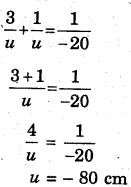The object has been placed at 80 cm from the mirror. The mirror is concave and the image formed is real and inverted.

Question 18.
Define power of a lens. What is its unit? One student uses a lens of focal length 50 cm and another of -50 cm. What is the nature of the lens and its power used by each of them?
The power of a lens is defined as the reciprocal of its focal length. It is represented by the letter P. The power P of a lens of focal length f is given by
p = $$\frac {1}{f}$$
The SI unit of power of a lens is ‘dioptre’.

The student who is having a lens of focal length 50 cm is having a convex lens and the other who is having a lens of focal length -50 cm, is having a concave lens.
The power of convex lens of
f = 50 cm or $$\frac {1}{2}$$ m
p = $$\frac {1}{f}$$
$$\frac{1}{1 / 2}=2 \mathrm{D}$$Question 19.
A student-focussed the image of a candle flame on a white scrcen using a convex lens. He noted down the position of the candle screen and the lens as under Position of candle = 12.0 cm
Position of convex lens = 50.0 cm
Position of the screen = 88.0 cm
(i) What is the focal length of the convex lens?
(ii) Where will the image be formed if he shifts the candle towards the lens at a position of 31.0 cm?
(iii) What will be the nature of the image formed if he further shifts the candle towa the lens?
(iv) Draw a ray diagram to show the formation of the image in case (iii) as said above.
Let (0, 0) is the origin on Cartesian co-ordinates from where the position of candle, convex lens and screen have been
considered(i) Now the distance between candle and convex lens is 50 – 12 = 38 cm. Now, using lhe lens formula :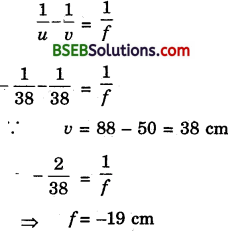∴ The focal length of the convex lens = 19 cm

(ii) If the candle is shifted towards the lens at a position of 31.0 cm, then distance of candle from the lens would be 50 – 31 = 19 cm, i.e., the candle will be at the focus of the convex lens. So, the image would be formed at infinity.

(iii) If the candle is further shifted towards the lens, then the position of the candle would be in between the optical centre and focus of the lens. Thus, the image formed would be virtual and erect.

(iv) The ray diagram to show the formation of the image in case (iii) as said above* is given below :